• 语音识别】基于语音分帧+端点检测+pitch提取+DTW算法歌曲识别matlab源码.md
• 语音识别】语音分帧+端点检测+pitch提取+DTW算法歌曲识别.mdmatlab代码
• 语音信号处理之语音分帧加窗，基于matlab仿真实验》》语音信号处理
• 一般来讲，语音信号的采样率是挺高的，而且认为语音信号在一定时间段的基本特性不会发生较大的...分帧通常有一定的交叠部分，就是移。移与长的比通常为0~1/2。 分帧就是通过加窗函数实现的，假设原始信号为...

《语音信号处理试验教程》（梁瑞宇等）的代码主要是Matlab实现的，现在Python比较热门，所以把这个项目大部分内容写成了Python实现，大部分是手动写的。使用CSDN博客查看帮助文件：

代码可在Github上下载busyyang/python_sound_open

一般来讲，语音信号的采样率是挺高的，而且认为语音信号在一定时间段的基本特性不会发生较大的变化，具有一定的短时平稳性。进行“短时分析”就行将信号分解成一段一段地来处理，每一段就叫一帧，大约10-30ms，也就是一秒钟大约33-100帧，对于通常10K的采样率来说，这样也能有一定的信息存在。分帧通常有一定的交叠部分，就是帧移。帧移与帧长的比通常为0~1/2。

分帧就是通过加窗函数实现的，假设原始信号为 s ( n ) s(n) ，窗函数为 w ( n ) w(n) ，那么分帧就是 s w ( n ) = s ( n ) ∗ w ( n ) s_w(n)=s(n)*w(n) ，窗函数需要满足1. 窗口两端不引起急剧变化，应该平滑过渡到0，2.在频域有较宽的3dB贷款以及较大的边带最大值。窗口的长度一般为10~20ms。有三种常见的窗函数为：

• 矩形窗
w ( n ) = { 1 , 0 ⩽ n ⩽ N − 1 0 , o t h e r s (矩形窗) w(n)=\left\{\begin{array}{ll} 1&,0\leqslant n \leqslant N-1\\ 0&,others \end{array}\right. \tag{矩形窗}

• 汉明窗

w ( n ) = { 0.54 − 0.46 cos ⁡ [ 2 π n / ( N − 1 ) ] , 0 ⩽ n ⩽ N − 1 0 , o t h e r s (汉明窗) w(n)=\left\{\begin{array}{ll} 0.54-0.46\cos[2\pi n/(N-1)]&,0\leqslant n \leqslant N-1\\ 0&,others \end{array}\right.\tag{汉明窗}

• 海宁窗

w ( n ) = { 0.5 ( 1 − cos ⁡ ( 2 π n / ( N − 1 ) ) ) , 0 ⩽ n ⩽ N − 1 0 , o t h e r s (汉明窗) w(n)=\left\{\begin{array}{ll} 0.5(1-\cos(2\pi n/(N-1)))&,0\leqslant n \leqslant N-1\\ 0&,others \end{array}\right.\tag{汉明窗}

其图形如下：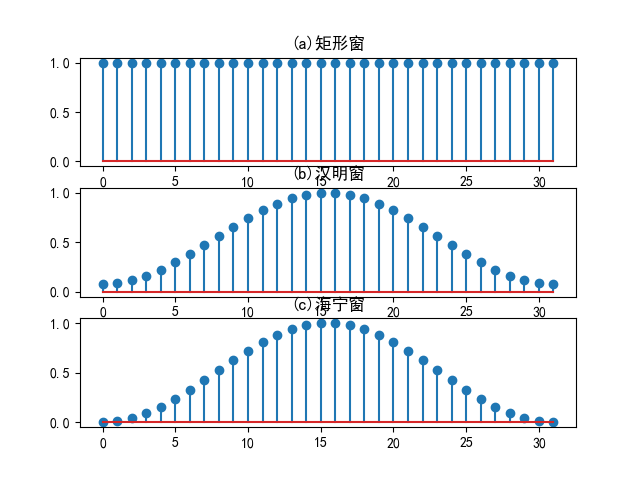import matplotlib.pyplot as plt
import numpy as np

plt.rcParams['font.family'] = ['sans-serif']
plt.rcParams['font.sans-serif'] = ['SimHei']

N = 32
nn = [i for i in range(N)]
plt.subplot(3, 1, 1)
plt.stem(np.ones(N))
plt.title('(a)矩形窗')

w = 0.54 - 0.46 * np.cos(np.multiply(nn, 2 * np.pi) / (N - 1))
plt.subplot(3, 1, 2)
plt.stem(w)
plt.title('(b)汉明窗')

w = 0.5 * (1 - np.cos(np.multiply(nn, 2 * np.pi) / (N - 1)))
plt.subplot(3, 1, 3)
plt.stem(w)
plt.title('(c)海宁窗')
# plt.show()
plt.savefig('images/window.png')
plt.close()

分帧操作，相当于将信号分解为若干个信号片段，并将片段与窗函数进行对应元素的乘法。

from scipy.io import wavfile
import numpy as np
import matplotlib.pyplot as plt

plt.rcParams['font.family'] = ['sans-serif']
plt.rcParams['font.sans-serif'] = ['SimHei']
plt.rcParams['axes.unicode_minus'] = False

def enframe(x, win, inc=None):
nx = len(x)
if isinstance(win, list):
nwin = len(win)
nlen = nwin  # 帧长=窗长
elif isinstance(win, int):
nwin = 1
nlen = win  # 设置为帧长
if inc is None:
inc = nlen
nf = (nx - nlen + inc) // inc
frameout = np.zeros((nf, nlen))
indf = np.multiply(inc, np.array([i for i in range(nf)]))
for i in range(nf):
frameout[i, :] = x[indf[i]:indf[i] + nlen]
if isinstance(win, list):
frameout = np.multiply(frameout, np.array(win))
return frameout

inc = 100
wlen = 200
en = enframe(data, wlen, inc)
i = input('起始帧(i):')
i = int(i)
tlabel = i
plt.subplot(4, 1, 1)
x = [i for i in range((tlabel - 1) * inc, (tlabel - 1) * inc + wlen)]
plt.plot(x, en[tlabel, :])
plt.xlim([(i - 1) * inc + 1, (i + 2) * inc + wlen])
plt.title('(a)当前波形帧号{}'.format(tlabel))

plt.subplot(4, 1, 2)
x = [i for i in range((tlabel + 1 - 1) * inc, (tlabel + 1 - 1) * inc + wlen)]
plt.plot(x, en[i + 1, :])
plt.xlim([(i - 1) * inc + 1, (i + 2) * inc + wlen])
plt.title('(b)当前波形帧号{}'.format(tlabel + 1))

plt.subplot(4, 1, 3)
x = [i for i in range((tlabel + 2 - 1) * inc, (tlabel + 2 - 1) * inc + wlen)]
plt.plot(x, en[i + 2, :])
plt.xlim([(i - 1) * inc + 1, (i + 2) * inc + wlen])
plt.title('(c)当前波形帧号{}'.format(tlabel + 2))

plt.subplot(4, 1, 4)
x = [i for i in range((tlabel + 3 - 1) * inc, (tlabel + 3 - 1) * inc + wlen)]
plt.plot(x, en[i + 3, :])
plt.xlim([(i - 1) * inc + 1, (i + 2) * inc + wlen])
plt.title('(d)当前波形帧号{}'.format(tlabel + 3))

# plt.show()
plt.savefig('images/en.png')
plt.close()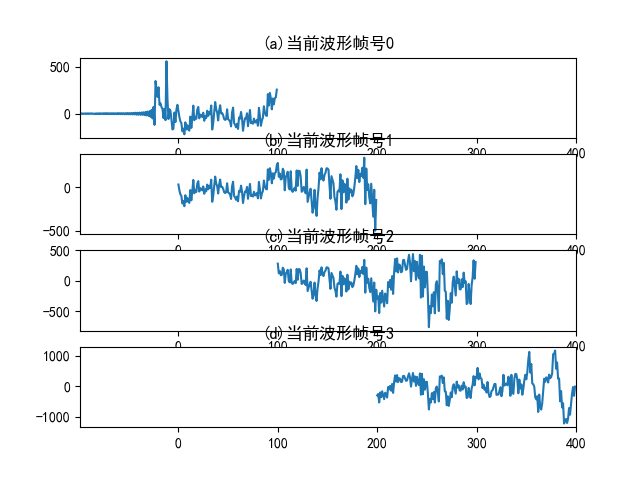另外，也可以直接使用numpy.hanning(N)来获得窗函数。其中N就是点数。

展开全文python 语音识别 信号处理
• 分帧一般而言语音处理的目的有两种：一种是对语音信号进行分析，提取特征参数，用于后续处理；提取的特征参数主要有语音的短时能量和平均幅度、短时平均过零率、短时自相关函数和短时平均幅度差函数等另一种是加工...

文章目录

原理

1.加窗

2.分帧

一般而言语音处理的目的有两种：

一种是对语音信号进行分析，提取特征参数，用于后续处理；

提取的特征参数主要有语音的短时能量和平均幅度、短时平均过零率、短时自相关函数和短时平均幅度差函数等

另一种是加工语音信号，

例如在语音增强中对含噪语音进行背景噪声抑制，以获得相对“干净”的语音；在语音合成方中需要对分段语音进行拼接平滑，获得主观音质较高的合成语音，这方面的应用同样是建立在分析并提取语音信号信息的基础上的。总之，语音信号分析的目的就在于方便有效地提取并表示语音信号所携带的信息。

根据所分析的参数类型，语音信号分析可以分成时域分析和变换域(频域、倒谱域)分析。其中时域分析方法是最简单、最直观的方法，它直接对语音信号的时域波形进行分析，

提取的特征参数主要有语音的短时能量和平均幅度、短时平均过零率、短时自相关函数和短时平均幅度差函数等。

原理

不论是分析怎样的参数以及采用什么分析方法，在按帧进行语音分析、提取语音参数之前，有一些经常使用的、共同的短时分析技术必须预先进行，如语音信号的数字化、预加重、加窗和分帧等，这些也是不可忽视的语音信号分析的关键技术。

1.加窗

窗函数可以加在时域或频域上，更多的是加在时域上

常用的窗函数

1.矩形窗

w ( n ) = { 1 , 0 ⩽ n ⩽ N − 1 0 , 其 他 w(n)=\left\{\begin{array}{ll}{1,} & {0 \leqslant n \leqslant N-1} \\ {0,} & {其他}\end{array}\right.w(n)={1,0,​0⩽n⩽N−1其他​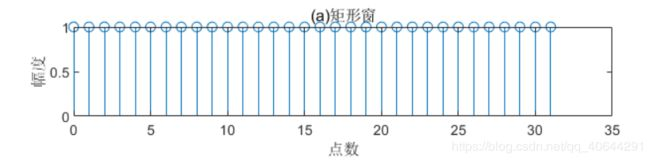2.汉明窗

w ( n ) = { 0.54 − 0.46 cos ⁡ [ 2 π n / ( N − 1 ) ] , 0 ⩽ n ⩽ N 0 ,  其他  w(n)=\left\{\begin{array}{ll}{0.54-0.46 \cos [2 \pi n /(N-1)],} & {0 \leqslant n \leqslant N} \\ {0,} & {\text { 其他 }}\end{array}\right.w(n)={0.54−0.46cos[2πn/(N−1)],0,​0⩽n⩽N其他​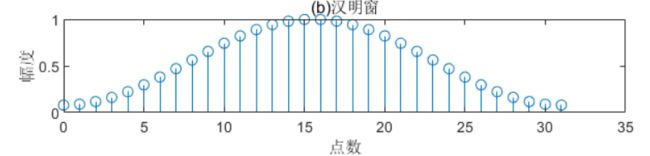3.海宁窗

w ( n ) = { 0.5 ( 1 − cos ⁡ [ 2 π n / ( N − 1 ) ] ) , 0 ⩽ n ⩽ N 0 ,  其他  w(n)=\left\{\begin{array}{ll}{0.5(1-\cos [2 \pi n /(N-1)]),} & {0 \leqslant n \leqslant N} \\ {0,} & {\text { 其他 }}\end{array}\right.w(n)={0.5(1−cos[2πn/(N−1)]),0,​0⩽n⩽N其他​上述窗函数对应的幅频响应曲线为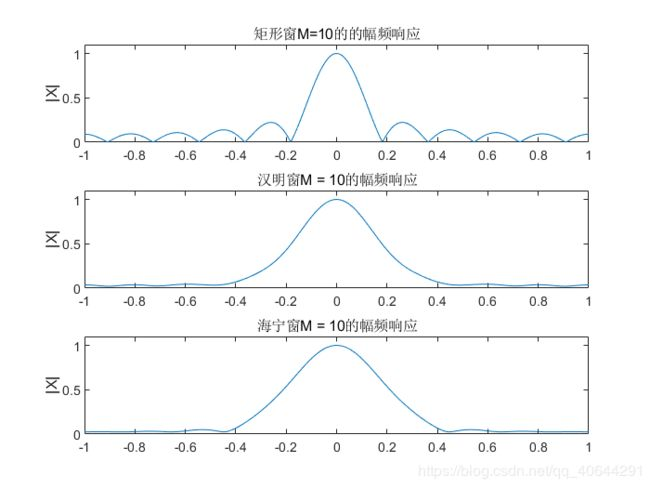注：矩形窗的主瓣宽度小于汉明窗，具有较高的频谱分辨率，但是矩形窗的旁瓣峰值较大，因此其频谱泄漏比较严重。

频谱泄漏( 对于频率为fs的正弦序列，它的频谱应该只是在fs处有离散谱。但是，在利用DFT求它的频谱时，对时域做了截断，结果使信号的频谱不只是在fs处有离散谱，而是在以fs为中心的频带范围内都有谱线出现，它们可以理解为是从fs频率上“泄漏”出去的，这种现象称 为频谱“泄漏”。 )

泄漏与窗函数频谱的两侧旁瓣有关，如果两侧旁瓣的高度趋于零，而使能量相对集中在主瓣，就可以较为接近于真实的频谱，为此，在时间域中可采用不同的窗函数来截断信号。

相比较，虽然汉明窗的主瓣宽度较宽，约大于矩形窗的一倍，但是它的旁瓣衰减较大，具有更平滑的低通特性，能够在较高的程度上反映短时信号的频率特性。

窗函数的选择(需要考虑其长度和形状)对短时分析参数的特性影响较大

所以选择合适的窗函数要考虑以下两个方面：

(1)窗口的形状

一个好的窗函数的标准是：在时域内语音波形乘以窗函数，要减小时间窗两端的坡度，使窗口边缘两端不引起急剧变化而平滑过渡到零，这样可以使截取出的语音波形缓慢降为零，减小语音帧的截断效应；在频域要有较宽的3dB带宽以及较小的边带最大值。

(2)窗口的长度

如果长度很大，则它等效于很窄的低通滤波器，语音信号通过时，反映波形细节的高频部分被阻碍，短时能量随时间变化很小，不能真实地反映语音信号的幅度变化：反之，长度太短时，滤波器的通带变宽，短时能量随时间有急剧的变化，不能得到平滑的能量函数。通常认为在一个语音帧内应包含1-7个基音周期。然而不同人的基音周期变化很大，从女性和儿童的2ms到老年男子的14 ms(即基音频率的变化范围为500-70 Hz)，所以N的选择比较困难。通常在10kHz取样频率下，N折中选择为100-200点较合适(即 10-20 ms 持续时

​ 三种窗函数的主瓣宽度B和第一旁瓣衰减A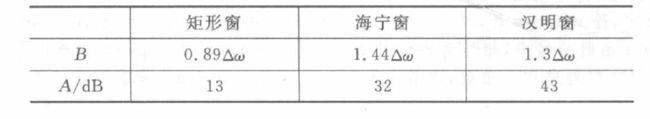△w是谱分析时的角频率分辨率。从表中可看出，矩形窗主瓣的宽度最窄，但第一旁瓣的衰减最小。也就是说，它的频谱泄漏要比另外两种窗函数大。在语音分析中，可根据不同的情况选择不同的窗函数。

2.分帧

贯穿于语音分析全过程的是“短时分析技术”

​ 语音信号从整体来看其特性及表征其本质特征的参数均是随时间而变化的，所以它是一个非平稳态过程，不能用处理平稳信号的数字信号处理技术对其进行分析处理。但是，由于不同的语音是由人的口腔肌肉运动构成声道某种形状而产生的响应，而这种口腔肌肉运动相对于语音频率来说是非常缓慢的，所以从另一方面看，虽然语音信号具有时变特性，但在一个短时间范围内(认为在10-30 ms的短时间内)，其特性基本保持不变即相对稳定，因而可以将其看作是一个准稳态过程，即语音信号具有短时平稳性。

​ 所以任何语音信号的分析和处理必须建立在“短时”的基础上，即进行“短时分析”，将语音信号分为一段一段来分析其特征参数，其中每一段称为一“帧”，帧长一般即取为10-30 ms。

对于整体的语音信号而言，

分析出的是由每一帧特征参数组成的特征参数时间序列。

分帧示意图如图所示。(其中N为帧长，M为帧移)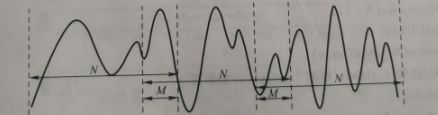一般每秒的帧数约为33-100帧，视实际情况而定。分帧虽然可以采用连续分段的方法，但一般要采用交叠分段的方法，这是为了使帧与帧之间平滑过渡，保持其连续性。前一帧和后一帧的交叠部分称为帧移。帧移与帧长的比值一般取为0~1/2

语音信号的分帧是采用可移动的有限长度窗口进行加权的方法来实现的。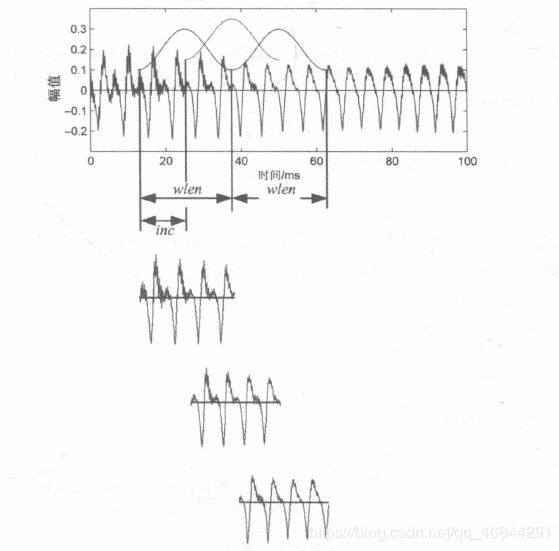设读入语音文件的数据存放在y中，y长为N，采样频率为fs，，取每帧长为wlen。在上图中给出的语音段正发生在两个音节间过渡区，基音正发生着变化。如果相邻两帧不重叠(图中的两个用实线表示的窗)，给出的基音可能有一个跳变。为了使其平稳过渡，在两帧之间再内插一帧或几帧，这样在相邻两帧之间就出现了重叠。后一帧对前一帧的位移量(简称为帧移)用inc表示，相邻两帧之间的重叠部分为overlap=wlen-inc。

对于长为N的语音信号分帧为

f n = ( N −  overlap  ) / i n c = ( N − w l e n + i n c ) / i n c = ( N − w l e n ) / i n c + 1 f n=(N-\text { overlap }) / i n c=(N-w l e n+i n c) / i n c \\ \quad\quad\quad\quad\quad\quad\quad\quad\quad\quad=(N-w l e n) / i n c+1fn=(N−overlap)/inc=(N−wlen+inc)/inc=(N−wlen)/inc+1

这样就可以进行分帧了。设分帧后的数组为yseg，其中y是列数据序列

数据将被分为fn帧,每一帧在数据y中开始的位置为

indf=(0:(nf-1))*inc+1

而其中每一帧的数据位置为1至wlen,设成

inds=1:wlen

其中,indf是一个fn×1的列向量;inds是一个1×wlen的行向量。

扩展这两个向量:

indf(:,ones(1,wlen)); %把indf扩展成fn x wlen的矩阵,每一列的数值都和原indf一样

inds(ones(fn,1),:); %把inds扩展成fn × wlen的矩阵,每一行的数值都和原inds一样

这个过程可以用repmat函数:

indf(:,ones(1,wlen))→repmat(indf,1,wlen);

inds(ones(fn,1),:)→repmat(inds,fn,1);

所以分帧过程可写为

yseg=y(repmat(indf,1,wlen)+repmat(inds,fn,1));

MATLB中常用enframe和segment函数来分帧，都是这个原理

fix(x) 函数用来用法说明：y=fix(x) 函数将x中元素零方向取整，得到y是一个整数数组。对于复数z，分别对实部和虚部取整

分帧函数如下

%分帧函数

function frameout=enframe(x,win,inc)

nx=length(x(:)); % 取数据长度

nwin=length(win); % 取窗长

if (nwin == 1) % 判断窗长是否为1，若为1，即表示没有设窗函数

len = win; % 是，帧长=win

else

len = nwin; % 否，帧长=窗长

end

if (nargin < 3) % 如果只有两个参数，设帧inc=帧长

inc = len;

end

nf = fix((nx-len+inc)/inc); % 计算帧数

frameout=zeros(nf,len); % 初始化

indf= inc*(0:(nf-1)).'; % 设置每帧在x中的位移量位置

inds = (1:len); % 每帧数据对应1:len

frameout(:) = x(indf(:,ones(1,len))+inds(ones(nf,1),:)); % 对数据分帧

if (nwin > 1) % 若参数中包括窗函数，把每帧乘以窗函数

w = win(:)'; % 把win转成行数据

frameout = frameout .* w(ones(nf,1),:); % 乘窗函数

end

下面对一个语音信号进行分帧(不使用窗函数的情况)，读取“我，到，北，京， 去”，选择起始帧号为70，显示出接下来的四帧

%语音分帧显示

%语音分帧显示

clc

clear all

wlen=200; inc=80; % 给出帧长和帧移

N=length(x); % 信号长度

time=(0:N-1)/Fs; % 计算出信号的时间刻度

signal=enframe(x,wlen,inc)'; % 分帧

i=input('请输入起始帧号(i):');

tlabel=i;

subplot 411;

% 画出第一帧时间波形

%tlabel-1)*inc+1:(tlabel-1)*inc+wlen 为起始帧第一帧的取值范围

plot((tlabel-1)*inc+1:(tlabel-1)*inc+wlen,signal(:,tlabel),'b');

%固定每一帧的长度

axis tight

xlim([(i-1)*inc+1 (i+2)*inc+wlen])

title(['(a)当前波形帧号：', num2str(i)]);

ylabel('幅值'); xlabel('帧长');

tlabel=i+1;

subplot 412;

% 画出第二帧时间波形

%(tlabel-1)*inc+1:(tlabel-1)*inc+wlen 为起始帧第二帧的取值范围

plot((tlabel-1)*inc+1:(tlabel-1)*inc+wlen,signal(:,tlabel),'b');

%固定每一帧的长度

axis tight

xlim([(i-1)*inc+1 (i+2)*inc+wlen])

title(['(b)当前波形帧号：', num2str(i+1)]);

ylabel('幅值'); xlabel('帧长');

tlabel=i+2;

subplot 413;

% 画出第三帧时间波形

plot((tlabel-1)*inc+1:(tlabel-1)*inc+wlen,signal(:,tlabel),'b');

%固定每一帧的长度

axis tight

xlim([(i-1)*inc+1 (i+2)*inc+wlen])

title(['(c)当前波形帧号：', num2str(i+2)]);

ylabel('幅值'); xlabel('帧长');

tlabel=i+3;

subplot 414;

% 画出第四帧时间波形

plot((tlabel-1)*inc+1:(tlabel-1)*inc+wlen,signal(:,tlabel),'b');

%固定每一帧的长度

axis tight

xlim([(i-1)*inc+1 (i+2)*inc+wlen])

title(['(d)当前波形帧号：', num2str(i+3)]);

ylabel('幅值'); xlabel('帧长');

运行结果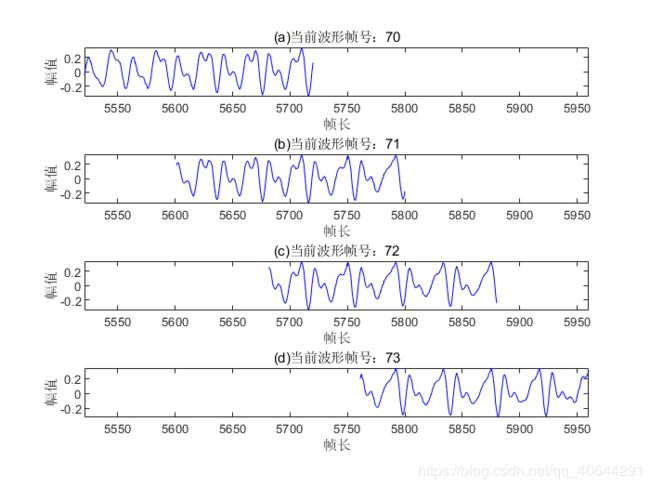展开全文• 语音信号分析（语音分帧）

万次阅读 多人点赞 2019-08-08 16:14:38
不管是进行什么参数分析以及采用什么分析方法，都需要一些预先的处理，如语音信号的数字化、语音信号的端点检测、预加重、加窗和分帧等，这些也是不可忽视的语音信号分析的关键技术。贯穿于语音分析全过程的是“短时...

语音分帧

不管是进行什么参数分析以及采用什么分析方法，都需要一些预先的处理，如语音信号的数字化、语音信号的端点检测、预加重、加窗和分帧等，这些也是不可忽视的语音信号分析的关键技术。贯穿于语音分析全过程的是“短时分析技术”。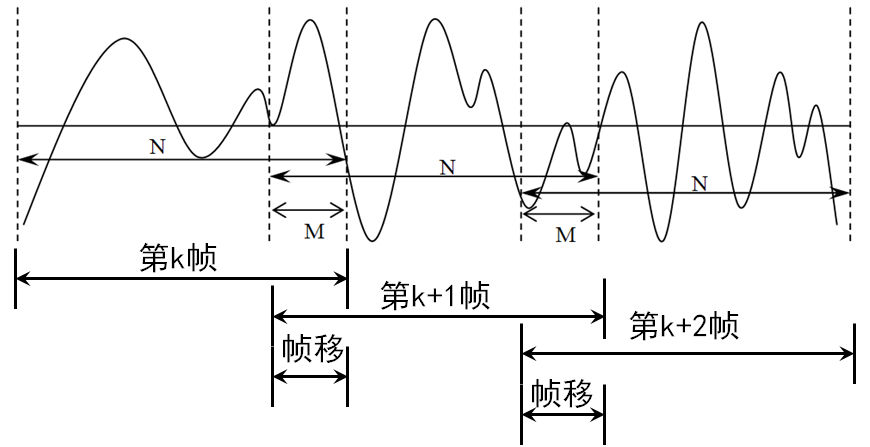分帧一般采用交叠分段的方法，是为了使帧与帧之间平滑过渡，保持其连续性。前一帧和后一帧的交叠部分称为帧移，帧移与帧长的比值一般取为0-1/2。

分帧是用可移动的有限长度窗口进行加权的方法来实现的，就是用一定的窗函数ω(n)来乘s(n)，从而形成加窗语音信号Sω(n)=s(n)×ω(n)

窗函数的要求：

1. 在时域因为是语音波形乘以窗函数，所以要减小时间窗两端的坡度，使窗口边缘两端不引起急剧变化而平滑过渡到零，这样可以使截取出的语音波形缓慢降为零，减小语音帧的截断效应；
2. 在频域要有较宽的3dB带宽以及较小的边带最大值。以典型的矩形窗和汉明窗为例进行比较如下。

常用窗函数的比较：

1. 窗函数的时域表达式：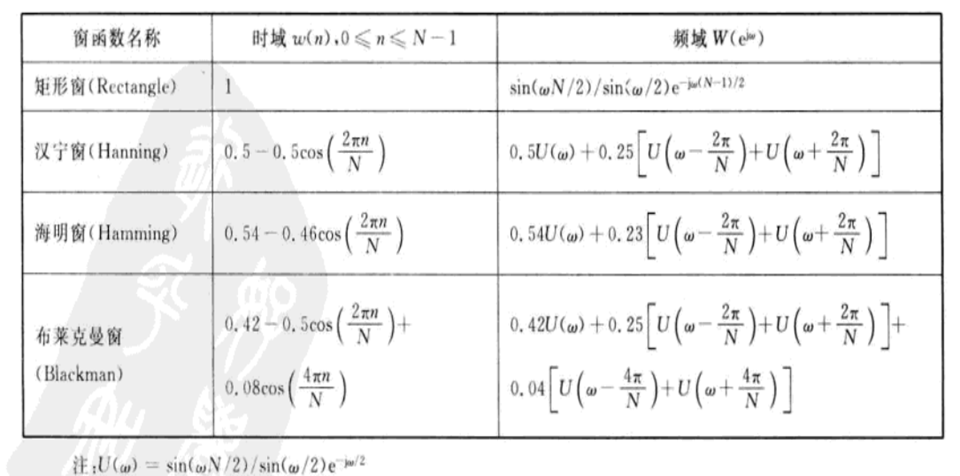2. 窗函数的时域对比：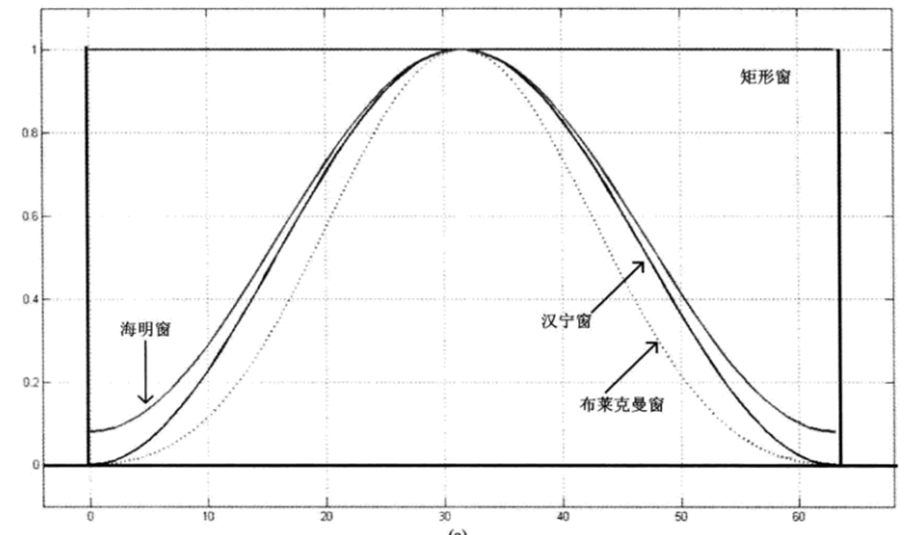3. 窗函数的频域对比：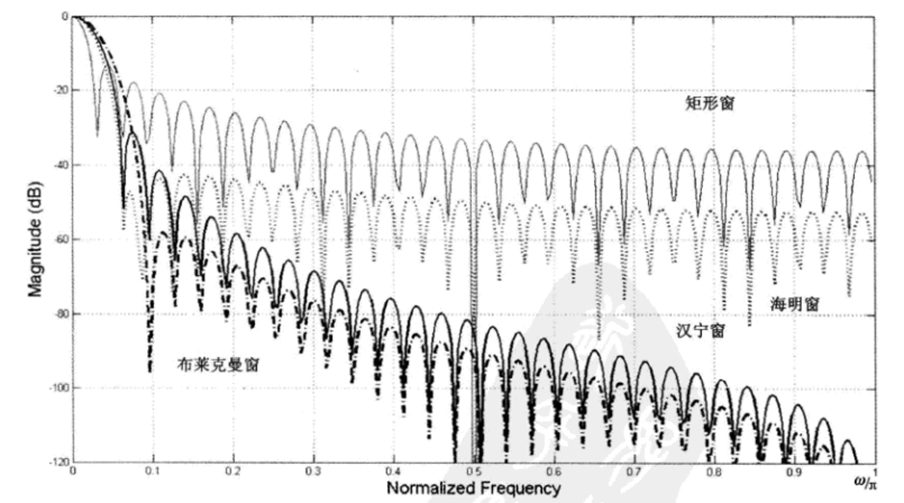窗函数的选择：

1. 加窗函数时，应使窗函数频谱的主瓣宽度应尽量窄，以获得高的频率分辨能力；
2. 旁瓣衰减应尽量大，以减少频谱泄漏（拖尾）；
3. 但二者矛盾需考虑折中，各种窗的差别主要在集中于主瓣的能量和分散在所有旁瓣的能量之比。
对语音信号的短时分析来说，窗口的形状是至关重要的。选用不同的窗口将使时域分析参数的短时平均能量的平均结果不同。

四种窗函数的频域特征：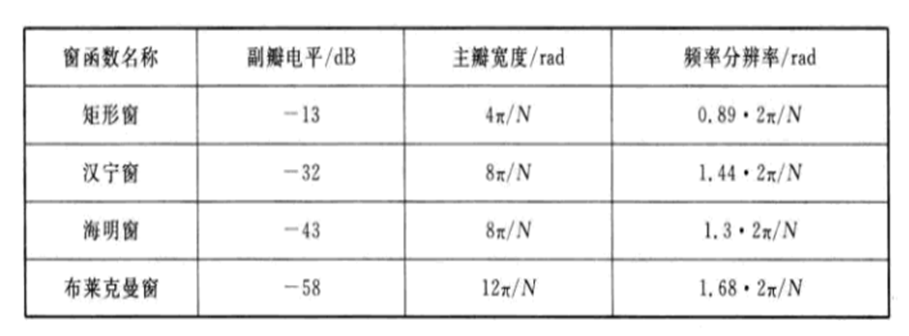我们可以看到汉明窗的主瓣宽度比矩形窗大1倍，即带宽约增加一倍，同时其带外衰减也比矩形窗大1倍多。矩形窗的谱平滑性能较好，但损失了高频成分，使波形细节丢失；而汉明窗则相反，从这一方面来看，汉明窗比矩形窗更为合适。汉明窗与汉宁窗对比，起始处小突变，但是副瓣平坦，很好的折中了突变小和平坦度，更适合。

窗口的长度：
采样周期Ts = 1/fs， 窗口长度N和频率分辨率Δf之间存在以下关系：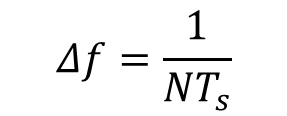当采样周期一定时，Δf随着窗口的宽度N的增大而减小，即频谱分辨率能够相应的提高，但是时间分辨率会降低；如果窗口取短，频率分辨率下降，而时间分辨率提高。因而二者是矛盾的，应该根据不同的需要选择合适的窗口长度。

有时窗口长度的选择，更重要的是要考虑语音信号的基音周期。通常认为在一个语音帧内应包含1～7个基音周期。然而不同人的基音周期变化很大，从女性和儿童的2ms到老年男子的14ms(即基音频率的变化范围为500～70Hz)，所以N的选择比较困难。通常在8kHz取样频率下，N折中选择为80～160点为宜(即10～20ms持续时间)。

经过上述的处理过程，语音信号就已经被分割成一帧一帧的加过窗函数的短时信号，然后再把每一个短时语音帧看成平稳的随机信号，利用数字信号处理技术来提取语音特征参数。在进行处理时，按帧从数据区中取出数据，处理完成后再取下一帧，等等，最后得到由每一帧参数组成的语音特征参数的时间序列

展开全文信号处理
• 语音分帧后的重组还原

千次阅读 2020-04-25 14:22:40
我们在对语音信号进行处理的时候经常需要对语音进行帧，而在帧的过程中帧与帧...在对语音信号处理后，我们会得到一个帧长为len_的处理后的语音帧，如果我们将这个整个帧重组原始语音的话，在处理后的第二帧里面...

我们在对语音信号进行处理的时候经常需要对语音进行分帧，而在分帧的过程中帧与帧之间会有一些重叠部分。在对每一帧处理之后我们需要将语音还原成原来的形式。下面详细说明语音分帧过程及如何还原重组语音。

如下图所示，在分帧过程中，帧长为len_，重叠长度为len1, 帧移为len2。在对语音信号处理后，我们会得到一个帧长为len_的处理后的语音帧，如果我们将这个整个帧重组原始语音的话，在处理后的第二帧里面有部分与第一帧重叠。为了避免这种重复带来的问题，如果语音长度恰好可以将帧长整除，我们在重组语音过程中每次只写入长度为len2的语音，即填充黄色区域对应的数据即可，其实可以发现语音帧有部分被丢弃了，这部分占很少的一部分对语音处理影响不大。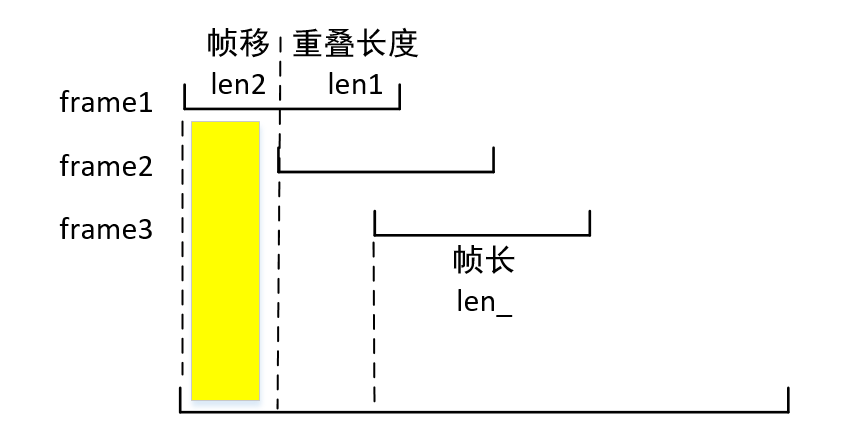下面以stft处理为例给出代码：

import librosa
from basic_functions import *

k = 0
nfft = 512
len_ = 240   # 帧长
len1 = 180   # 重叠长度
len2 = 60    # 帧移
frame_nums = len(x) // len2

xfinal = np.zeros(len(x))

for i in range(frame_nums):
frame = x[k:k + len_]
spectrum = librosa.stft(frame, window="hann",n_fft=nfft, win_length=nfft, hop_length=nfft)
xi = librosa.istft(spectrum, window="hann", win_length=nfft, hop_length=nfft, length=len_)

xfinal[k:k + len2] =  xi[0:len2]
k = k + len2

displaySpeech(x - xfinal, 8000)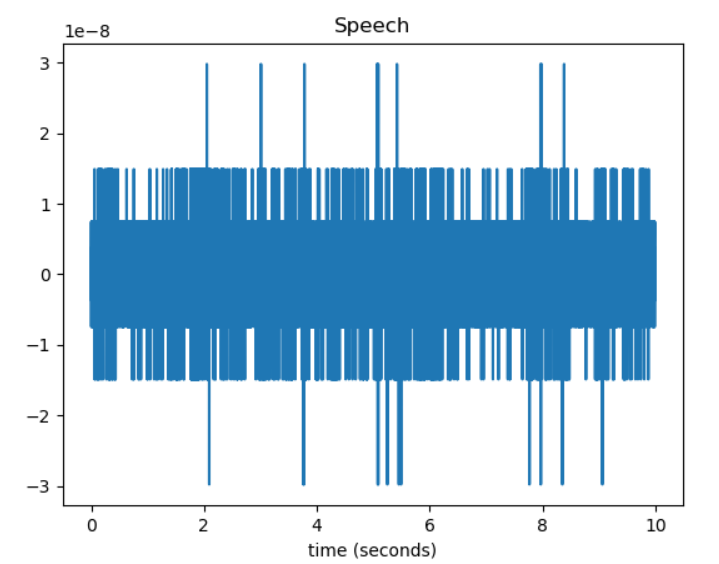展开全文• 语音分帧加窗

千次阅读 2019-03-31 15:44:17
分帧（chunk） 傅里叶变换要求输入的信号的平稳的。 语音信号在宏观上是不平稳的，在微观上是平稳的，具有短时平稳性（10—30ms内可以认为语音信号近似不变），这个就可以把语音信号分为...
• 语音分帧函数

2015-07-16 10:58:44
使用matlab进行语音分帧处理函数，是.M文件
• 语音信号处理：短时过零率，语音分帧，短时功率密度谱，短时能量，小波去噪
• 语音加窗分帧

2013-03-14 16:40:11
该段代码实现了语音的录入（包括采样）并进行加窗分帧，最后表示出原录入语音和加窗分帧后的语音对比图
• 用matlab书写的语音分帧函数，主要用于语音识别方面
• 语音分帧的帧移怎样理解

千次阅读 2020-10-23 13:17:16
当时不太明白，为啥分帧之后还要重叠取语音信号。在知乎上看到一篇文章，讲的不错：语音分帧的理解 对音频信号分帧，在较短的时间内，认为信号平稳信号，对其做傅里叶变换分析其频谱特征。分成的小信号片段，需要乘...
• 本文主要实现使用python编程实现对我上一个博客写的python编程读取wav文件数据得到的语音数据进行分帧，同时也编程实现将分帧后的数据还原为原始语音数据。 第一部分主要实现用python编程实现对语音数据进行分帧。 ...
• 在用Matlab进行语音分析时，通常会用读入的数据进行分帧处理。在分帧中，往往设置在相邻两之间有一部分重叠。 其原因是：语音信号是时变的，在短时范围内特征变化较小，所以做为稳态来处理；但超出这短时范围...语音处理 语音分析 Matlab 语音信号 音频处理
• 自己写的关于语音分帧，加窗，去噪，端点检测方面的程序，可成功调试-Wrote it myself on the voice sub-frames, plus windows, de-noising, endpoint detection procedures can be successfully debug
• MATLAB实现语音信号分帧

千次阅读 2020-10-27 10:39:05
语音文件的数据存放在y中，y的长度为N，采样频率为fs，取每长为wlen，后一对前一的位移量用inc表示，相邻两之间的重叠部分overlap=wlen-inc。 二、分帧函数调用 f=enframe（x，win,inc） x是数据，win是...
• 加窗分帧：时域加窗，点乘；频域卷积，减少泄露 解释：语音信号是时变信号，浊音的基音周期、清浊音信号幅度和声道参数均随时间变化，发声器官的惯性运动，可以认为具有短时平稳性。长10~30ms，交叠分段方式，...matlab 信号处理 语音识别
• python 语音加窗分帧

千次阅读 2019-04-28 20:22:44
from__future__importdivision fromscikits.talkboximportsegment_axis importnumpy as np importsoundfile as sf defreadwav(fn): signal, sampleRate=sf.read(fn) ...signal-=np.mean(...
• python 语音数据进行分帧

千次阅读 2019-08-09 09:52:23
# -*- coding: utf-8 -*- from scipy.io import wavfile import numpy as np fs, data = wavfile.read(r'./bluesky3.wav') nx = len(data) ...nframe = int((nx - wlen + inc) / inc) # 计算数...
• 那么一有多长呢？长要满足两个条件： 从宏观上看，它必须足够短来保证内信号是平稳的。 前面说过，口型的变化是...语音的基频，男声在 100 赫兹左右，女声在 200 赫兹左右，换算成周期就是 10 毫秒和 5 毫秒。.
• 分帧是为了将无限长的语音信号，分成一段一段的，因为语音信号具有短时平稳性，方便处理，加窗是为了使分帧后的语音信号更加平稳。窗函数主要有矩形窗和汉明窗。加窗主要是为了使时域信号似乎更好地满足FFT处理的...matlab 语音信号
• 语音信号处理 预加重 一阶高通滤波器 分帧加窗三种窗函数比较 端点检测 双门限 频谱分析 录音程序也有，用户可自己录音，进行处理
• 语音信号的分帧、加窗处理

千次阅读 2019-11-09 15:24:47
语音信号是一个准稳态的信号，若把它分成较短的，每中可将其看做稳态信号，可用处理稳态信号的...将语音信号分帧后，需要对每一信号进行分析处理。窗函数一般具有低通特性，加窗函数的目的是减少频域中的泄漏。
• 再对原语音信号进行分帧，并使用了三种窗函数（矩形窗，海宁窗，海明窗）对其中的一进行加窗，查看结果。 注：本文所用的matlab版本为matlab 2017a 首先读取音频文件： [x1,fs]=audioread('lantian.wav'); %读取......